JOINProblem Statement
Contest: 2012 TCO Marathon Round 2
Problem: TwistedGame

### Problem Statement

Twisted is a game played by arranging square tiles in cells of an infinite grid. Each side of the tile is divided into three equal parts by two contacts. Each tile has exactly 4 pieces of wire on it, each piece connects two distinct contacts on the sides of the tile, and each contact has exactly one piece of wire connected to it. Whenever two tiles get placed in vertically or horizontally adjacent cells, matching contacts on the side they share get connected and form a chain of wire.

Below you can find several examples of tiles: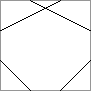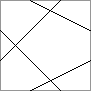You will be given at most N tiles, one by one. You need to place each tile on the board. The first tile is placed automatically in a cell (N, N), without rotation. You have to choose the cells to place each of the following tiles and how much you want to rotate it when placing. The second tile must be placed in a cell adjacent to the cell of the first tile. When the second tile is placed, the main chains are defined as chains of wire which go through one of the contacts on the side shared by the first and the second tiles. The active contacts are the contacts on both ends of the main chains.

After the second tile is placed, there can be 1 or 2 main chains and 0, 2 or 4 active contacts. Consider examples below (main chains are drawn in red):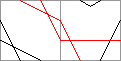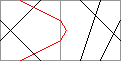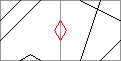Each tile after the second one must extend one of the main chains, i.e., one of the wires on it must become connected to one of the active contacts: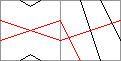===>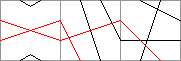It's also possible to extend more than one main chain in one move: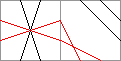===>If a new tile is placed so that a wire on it connects an active contact to another chain of wires, this chain becomes part of the main chain as well, and its other end becomes active, while the previously active contact gets deactivated:===>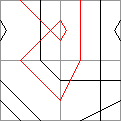As can be noticed on some of the pictures, as game progresses, two main chains can become merged into one and also each of the main chains can get transformed into a closed loop (that can't be further extended). The game ends either when all N tiles are placed or when there are no active contacts anymore (because all chains on the board are looped). An example of such situation is shown below: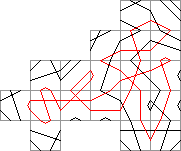Your goal is to achieve as large length of a main chain as possible in the end of the game. The length of a chain is just the number of wires in it, i.e., each single wire is assumed to have a length of 1 disregarding of how it is located within its cell.

Your code should implement two methods, as described below:
• int init(int N, int[] firstTile). This method is called once per test case to give you the total number of tiles and the first tile which is placed at (N, N) without rotation. You can return any int from this method, it will be ignored. The value of N will be 10000 in all test cases except the first 3 examples.

• String placeTile(int[] tile). This method is called on each move to give you the next tile to be placed. In both methods a tile is described as a int[] with exactly 8 elements in the following way. The contacts on the sides of the tile are indexed like this:

```       0 1
. .
7.   .2
6.   .3
. .
5 4```

And pairs of contacts with indices tile[2*i] and tile[2*i+1], 0 <= i < 4, are connected with a wire.

Each tile supplied to your solution (including the first one) will be generated as a random permutation of integers between 0 and 7, inclusive.

This method must return the coordinates (row and column) of the cell at which this tile will be placed and the rotation of the tile. Rows are numbered top to bottom and columns are numbered left to right. The rotation of 0 means that the tile won't be rotated, values 1, 2 and 3 mean rotation by 90, 180 and 270 degrees in clockwise direction. Your return must be formatted as "ROW COL ROT" (quotes for clarity only).

If you think you're running out of time or can't find a way to place the tile, you can return "GIVE UP". In this case this tile won't be placed, and your score will be calculated based on the current state of the board. Invalid returns (as well as other exceptional situations like exceeding time/memory limit or crashing) are also treated as giving up.

The cases in which it's impossible to place a tile due to lack of active contacts will be detected by the tester, and your solution won't be called.

Your score for a single test case will be equal to the length of the largest main chain present on the board once the game was stopped. Your overall score will be the arithmetic average of your individual scores over all test cases.

An offline tester and a separate visualization tool are available.

### Definition

 Class: TwistedGame Method: init Parameters: int, int[] Returns: int Method signature: int init(int N, int[] firstTile) Method: placeTile Parameters: int[] Returns: String Method signature: String placeTile(int[] tile) (be sure your methods are public)

### Notes

-The memory limit is 1024 MB and the time limit is 10 seconds (which includes only time spent in your code).
-There is no explicit code size limit. The implicit source code size limit is around 1 MB (it is not advisable to submit codes of size close to that or larger). Once your code is compiled, the binary size should not exceed 1 MB.
-The compilation time limit is 30 seconds. You can find information about compilers that we use and compilation options here.
-There are 10 example test cases and 100 full submission test cases.

### Examples

0)

```Seed = 1
N = 10
```
1)

```Seed = 2
N = 100
```
2)

```Seed = 3
N = 1000
```
3)

```Seed = 4
N = 10000
```
4)

```Seed = 5
N = 10000
```
5)

```Seed = 6
N = 10000
```
6)

```Seed = 7
N = 10000
```
7)

```Seed = 8
N = 10000
```
8)

```Seed = 9
N = 10000
```
9)

```Seed = 10
N = 10000
```

This problem statement is the exclusive and proprietary property of TopCoder, Inc. Any unauthorized use or reproduction of this information without the prior written consent of TopCoder, Inc. is strictly prohibited. (c)2010, TopCoder, Inc. All rights reserved.